This post explains how to build an area over flexible baseline chart using Python and the Plotly library. This combination of line and area plots is particularly suitable to show deviations from a reference or baseline that can be flexible.

This page showcases the work of Jan Kühn published as a web app called MeteoHist. It can be used to create Area over flexible baseline charts for temperature and precipitation data. The code has been published on GitHub.

The simplified example presented here, shows step by step how to use historical temperature data from open-meteo.com to compare a given year to the mean and percentile values of a reference period. The plot is created first using the `Matplotlib` library in `Python` and then `Plotly`, which allows to add interaction.

## Import modules

First, we import the modules we need.

``````import datetime as dt
from calendar import isleap

import matplotlib as mpl
import matplotlib.dates as mdates
import matplotlib.pyplot as plt
import numpy as np
import pandas as pd
import plotly.graph_objects as go
import requests
import seaborn as sns
from matplotlib import ticker
from plotly.express.colors import sample_colorscale
from statsmodels.nonparametric.smoothers_lowess import lowess``````

## Get the data

For this plot, we use data from the Historical Weather API provided by open-meteo.com. It is based on data from reanalysis datasets of the European Union's Copernicus Programme. The data can be downloaded using a simple API call.

In this example, we will be using coordinates of Addis Ababa, Ethiopia and mean temperature two meters above the ground to compare the values of 2022 to the reference period 1961-1990.

``````# Define values to get mean temperature data from API
lat, lon = 9.035845, 38.752406
date_start = "1961-01-01"
date_end = "2022-12-31"
metric_data = "temperature_2m_mean"
units = "celsius"

# Construct the API URL
url = (
"https://archive-api.open-meteo.com/v1/archive?"
f"latitude={lat}&longitude={lon}&"
f"start_date={date_start}&end_date={date_end}&"
f"daily={metric_data}&timezone=auto"
f"&temperature_unit={units}"
)

# Get the data from the API
data = requests.get(url, timeout=30)

# Create new Dataframe from column "daily"
df_raw = pd.DataFrame(
{"date": data.json()["daily"]["time"], "value": data.json()["daily"][metric_data],}
)

# Convert date column to datetime
df_raw["date"] = pd.to_datetime(df_raw["date"])``````

Have a look at the data:

``df_raw``
date value
0 1961-01-01 12.3
1 1961-01-02 13.4
2 1961-01-03 13.3
3 1961-01-04 12.8
4 1961-01-05 12.8
... ... ...
22640 2022-12-27 14.8
22641 2022-12-28 13.5
22642 2022-12-29 12.9
22643 2022-12-30 12.7
22644 2022-12-31 12.6

22645 rows × 2 columns

## Transform data

Next, we have to apply some transformations. For the plot we need a dataframe with one row for each day of the year and columns holding the following values:

• `dayofyear`: Day of the year (integer from 1 to 365, without leap days)
• `p05`: (Smoothed) 5th percentile of the reference period data
• `mean`: (Smoothed) mean of the reference period data
• `p95`: (Smoothed) 95th percentile of the reference period data
• `2022`: Temperature value for the year we compare (in this case 2022)
• `2022_diff`: Difference of the current year's value to the mean
• `2022_alpha`: Opacity values (0.6 if year's value is between p05 and p95, else 1)
• `date`: Datetime object of the day
``````# Define year and reference period
year = 2022
reference_period = (1961, 1990)

# Create a copy of the raw dataframe
df_f = df_raw.copy()

# Add column with day of year
df_f["dayofyear"] = df_f["date"].dt.dayofyear

# Remove all Feb 29 rows to get rid of leap days
df_f = df_f[~((df_f["date"].dt.month == 2) & (df_f["date"].dt.day == 29))].copy()

# Adjust "dayofyear" values for days after February 29th in leap years
df_f["dayofyear"] = df_f["dayofyear"].where(
~((df_f["date"].dt.month > 2) & (df_f["date"].dt.is_leap_year)),
df_f["dayofyear"] - 1,
)

# Reset index
df_f.reset_index(drop=True, inplace=True)

# Filter dataframe to reference period
data = df_f[df_f["date"].dt.year.between(*reference_period)].copy()

# Group by day of year and calculate 5th percentile, mean, and 95th percentile
data = (
data.groupby("dayofyear")["value"]
.agg(
[
("p05", lambda x: np.nanpercentile(x, 5)),
("mean", "mean"),
("p95", lambda x: np.nanpercentile(x, 95)),
]
)
.reset_index()
)

# Add smoothing using LOWESS (Locally Weighted Scatterplot Smoothing)
# to the 5th percentile, mean, and 95th percentile
for col in ["p05", "mean", "p95"]:
smoothed_values = lowess(
data[col],
data["dayofyear"],
is_sorted=True,
# Fraction of data used when estimating each y-value
# 1/12 roughly equals one month (= a lot of smoothing)
frac=1 / 12,
)

data[col] = smoothed_values[:, 1]

# Add column with year's value
data[f"{year}"] = df_f[df_f["date"].dt.year == year]["value"].reset_index(drop=True)

# Add column that holds the difference between the year's value and the mean
data[f"{year}_diff"] = data[f"{year}"] - data["mean"]

# If given year is a leap year, this has to be accounted for
# by adding 1 to the day of year after February 28th
dayofyear = data["dayofyear"]

if isleap(year):
dayofyear = data["dayofyear"].where(
data["dayofyear"] < 60, other=data["dayofyear"] + 1
)

data["date"] = dayofyear.apply(
lambda x: dt.datetime(year, 1, 1) + dt.timedelta(days=x - 1)
)``````

Have a look at the dataframe:

``data``
dayofyear p05 mean p95 2022 2022_diff date
0 1 11.252559 13.130396 14.814301 16.6 3.469604 2022-01-01
1 2 11.322329 13.175637 14.847490 17.5 4.324363 2022-01-02
2 3 11.392410 13.220881 14.880949 16.0 2.779119 2022-01-03
3 4 11.462678 13.266079 14.914659 15.1 1.833921 2022-01-04
4 5 11.533004 13.311186 14.948560 15.3 1.988814 2022-01-05
... ... ... ... ... ... ... ...
360 361 11.675899 13.272582 14.799901 14.8 1.527418 2022-12-27
361 362 11.695305 13.285921 14.816865 13.5 0.214079 2022-12-28
362 363 11.714921 13.299838 14.834247 12.9 -0.399838 2022-12-29
363 364 11.734664 13.314251 14.852072 12.7 -0.614251 2022-12-30
364 365 11.754446 13.329053 14.870351 12.6 -0.729053 2022-12-31

365 rows × 7 columns

## Create the plot using Matplotlib

Now that we transformed the data, we can start creating the plot. First, we define a figure with some basic settings.

``````# Set seaborn style to white with horizontal grid lines
sns.set_style("white")

# Define some basic settings for the plot
plt.rcParams["figure.dpi"] = 100
mpl.rcParams["font.family"] = "sans-serif"
mpl.rcParams["font.sans-serif"] = "Lato"
mpl.rcParams["axes.labelsize"] = 11
mpl.rcParams["xtick.labelsize"] = 11
mpl.rcParams["ytick.labelsize"] = 11

# Create a new figure and axis
fig, axes = plt.subplots(figsize=(10, 7))

plt.suptitle(
f"Mean temperature in Addis Ababa, Ethiopia {year}",
fontsize=24,
fontweight="bold",
x=1,
ha="right",
)
plt.title(
(
f"Compared to historical daily mean temperatures "
f"({reference_period}-{reference_period})"
),
fontsize=14,
fontweight="normal",
x=1,
ha="right",
)

left=0,
right=1,
top=0.87,
)

# Remove the borders of the plot
axes.spines["top"].set_visible(False)
axes.spines["bottom"].set_visible(False)
axes.spines["right"].set_visible(False)
axes.spines["left"].set_visible(False)

axes.grid(axis="y", color="0.9", linestyle="-", linewidth=1)``````

### Plot percentile lines and area between them

First we add the 5th and 95th percentile lines to the plot and fill the area between them. Since Matplotlib provides the `zorder` parameter, we can make sure that the percentile lines are plotted above the other elements.

``````# Plot the historical mean for each day of the year
axes.plot(
data.index,
data["mean"],
color="black",
zorder=10,
)

# Plot area between p05 and p95
percentiles = ["05", "95"]

axes.fill_between(
data.index,
data[f"p{percentiles}"],
data[f"p{percentiles}"],
color="#f8f8f8",
)

# Plot the percentile lines
for percentile in percentiles:
# Add dashed line for percentile
axes.plot(
data.index,
data[f"p{percentile}"],
label=f"{percentile}th Percentile",
color="black",
linestyle="dashed",
linewidth=0.5,
zorder=9,
)
# Place a label on the line
axes.text(
data.index[-1],
data[f"p{percentile}"].iloc[-1],
f"P{percentile}",
horizontalalignment="left",
verticalalignment="center",
color="black",
)

fig
``````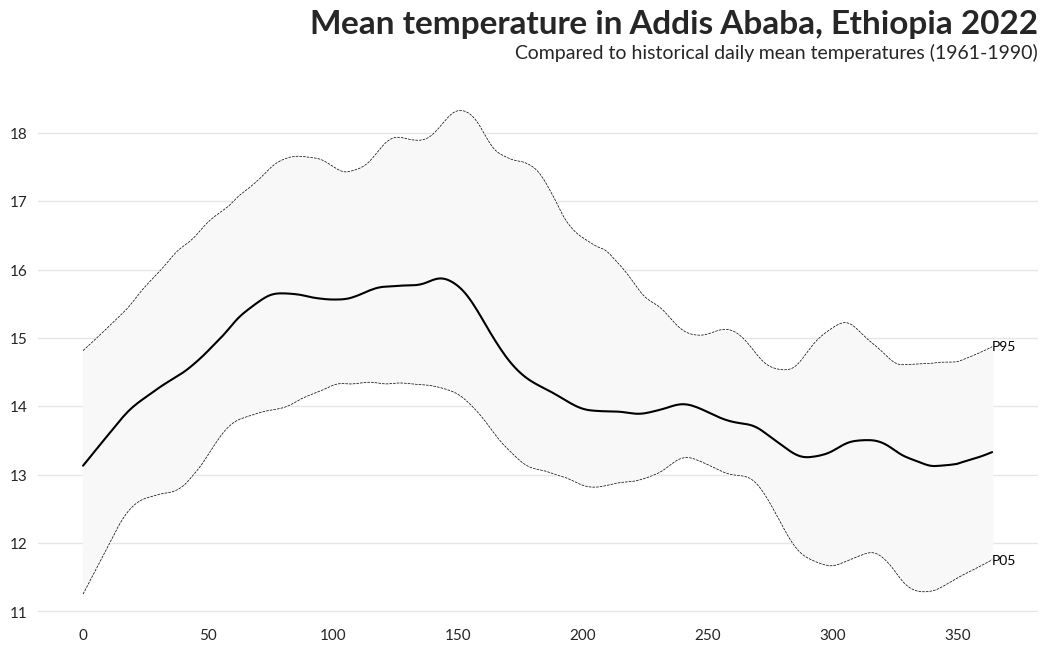### Plot the difference between the year's value and the long-term mean

Next we add the daily values for the selected year. Of course we want the peaks to be displayed in a color, that represents the amount of deviation from the mean value. So we create two colorscales to be used: One for values above the mean and one for values below. We define a function to calculate these colorscales and combine them into one array.

#### Function to calculate colorscales

``````def get_colorscale_mpl(diff: np.ndarray) -> np.ndarray:
"""
Calculate colorscale for a given series of values.
"""

# Create masks for above and below mean

# Get absolute value of difference
diff = abs(diff)

# Create array of zeros with same shape as diff
diff_norm = np.zeros_like(diff)

# Calculate min and max for values above the mean and normalize to 0-1

# Calculate min and max for values below the mean and normalize to 0-1

# Choose a colormap
colormap_above = plt.get_cmap("YlOrRd")
colormap_below = plt.get_cmap("YlGnBu")

# Create an empty array of the length of diff_norm but with 4 channels
colors = np.zeros((len(diff_norm), 4))

# Sample colors from colormaps, using normalized values

return colors``````

#### Plot the values

``````# Get colorscale using the function defined before
colors = get_colorscale_mpl(data[f"{year}_diff"])

# Set opacity to 0.6 for values between p05 and p95, otherwise 1
opacity = np.where(
(data[f"{year}"] >= data["p05"]) & (data[f"{year}"] <= data["p95"]), 0.6, 1
)

# Plot the area above and below the mean separately
for method in ["above", "below"]:
# Create mask to filter for values above mean and reverse for below
if method == "below":

for i in range(len(data.index) - 1):
# Get values for current method, leave rest as NaN
mean = data["mean"].to_numpy()

# To avoid mix-ups between colorscales, set tomorrow's value to mean
# if the area switches between above and below the mean
if (values[i] > mean[i]) ^ (values[i + 1] > mean[i + 1]):
values[i + 1] = mean[i + 1]

# Plot area between mean and year's value
axes.fill_between(
x=data.index[i : i + 2],
y1=values[i : i + 2],
y2=mean[i : i + 2],
color=colors[i],
alpha=opacity[i],
edgecolor="none",
zorder=8,
)

fig
``````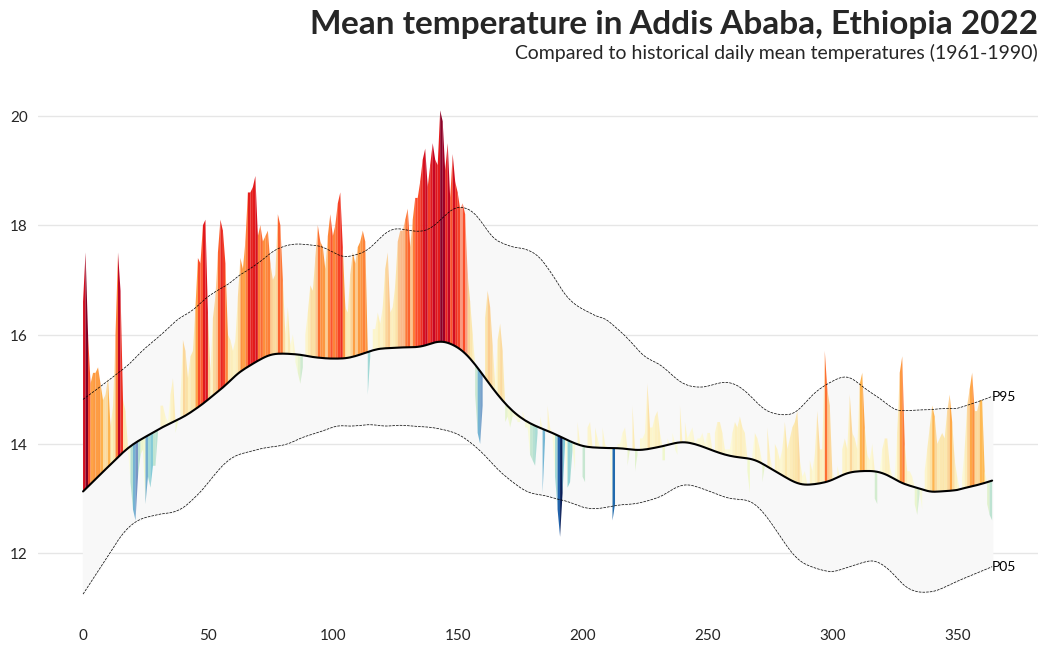### Add alternating background color for months

``````# Define dict with first and last day of each even month
months_with_days = [(32, 59), (91, 120), (152, 181), (213, 243), (274, 304), (335, 365)]

# Add background color for these months
for month_span in months_with_days:
axes.axvspan(
month_span,
month_span,
facecolor="#f8f8f8",
edgecolor=None,
alpha=0.3,
zorder=0,
)

fig
``````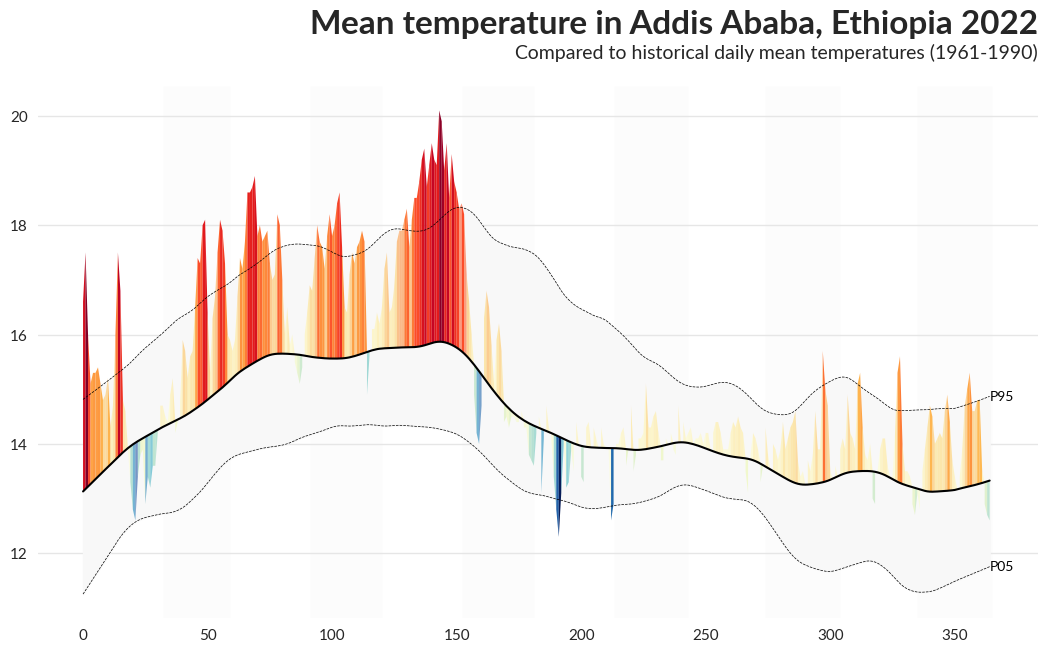We change the x-axis to display month names instead of numbers and add "°C" to the y-axis tick labels.

``````# 16 is a slight approximation since months differ in number of days.
axes.xaxis.set_major_locator(mdates.MonthLocator())
axes.xaxis.set_minor_locator(mdates.MonthLocator(bymonthday=16))
axes.xaxis.set_major_formatter(ticker.NullFormatter())
axes.xaxis.set_minor_formatter(mdates.DateFormatter("%b"))

for tick in axes.xaxis.get_minor_ticks():
tick.tick1line.set_markersize(0)
tick.tick2line.set_markersize(0)
tick.label1.set_horizontalalignment("center")

# Make the first and last x-axis label invisible
if axes.get_xticklabels(minor=True):
axes.get_xticklabels(minor=True).set_visible(False)
axes.get_xticklabels(minor=True)[-1].set_visible(False)

# Add °C to y-axis ticks
ticks = axes.get_yticks()
labels = [f"{int(x)}°C" for x in ticks]
axes.set_yticks(ticks, labels)

fig
``````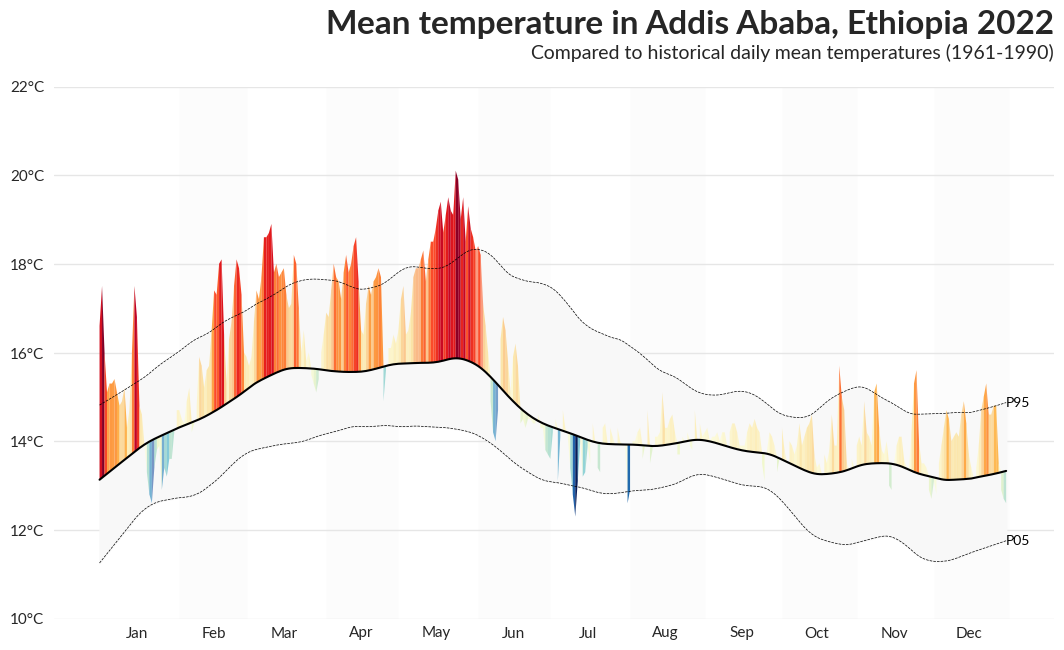### Add annotations and data source

To make the chart easier to understand, we add annotations for mean line and percentile area and the data source.

``````# Add annotation for mean line, with arrow pointing to the line
axes.annotate(
f"Mean Temperature\n{reference_period}-{reference_period}",
xy=(74, data["mean"].iloc),
xytext=(105, 12),
arrowprops={
"arrowstyle": "-",
"facecolor": "black",
"edgecolor": "black",
"shrinkB": 0,  # Remove distance to mean line
},
horizontalalignment="center",
verticalalignment="center",
color="black",
zorder=10,
)

# Add annotation for mean line, with arrow pointing to the line
axes.annotate(
"90% of reference period\nvalues fall within the gray area",
xy=(196, 16),
xytext=(258, 19),
arrowprops={
"arrowstyle": "-",
"facecolor": "black",
"edgecolor": "black",
"shrinkB": 0,  # Remove distance to mean line
},
horizontalalignment="center",
verticalalignment="center",
color="black",
zorder=10,
)

fig.text(
1,
0,
(
"Data: open-meteo.com, OSM  "
"Graph: Jan Kühn, https://yotka.org"
),
ha="right",
va="bottom",
fontsize=8,
alpha=0.5,
)

fig
``````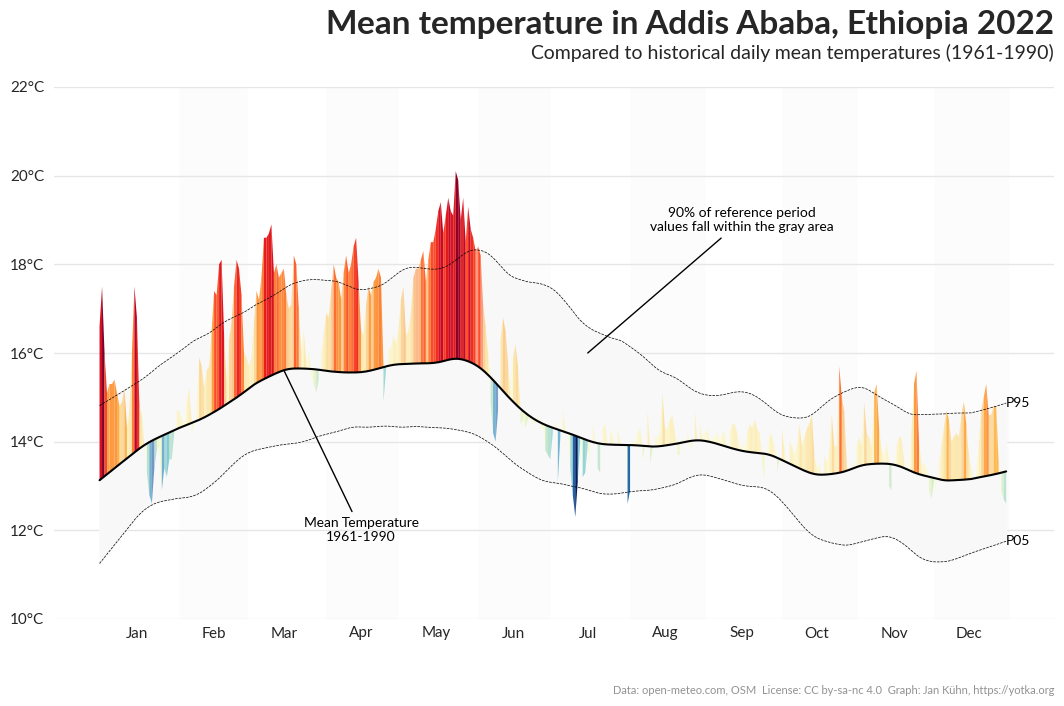### Complete code for the plot

``````# Set seaborn style to white with horizontal grid lines
sns.set_style("white")

# Define some basic settings for the plot
plt.rcParams["figure.dpi"] = 100
mpl.rcParams["font.family"] = "sans-serif"
mpl.rcParams["font.sans-serif"] = "Lato"
mpl.rcParams["axes.labelsize"] = 11
mpl.rcParams["xtick.labelsize"] = 11
mpl.rcParams["ytick.labelsize"] = 11

# Create a new figure and axis
fig, axes = plt.subplots(figsize=(10, 7))

plt.suptitle(
f"Mean temperature in Addis Ababa, Ethiopia {year}",
fontsize=24,
fontweight="bold",
x=1,
ha="right",
)
plt.title(
(
f"Compared to historical daily mean temperatures "
f"({reference_period}-{reference_period})"
),
fontsize=14,
fontweight="normal",
x=1,
ha="right",
)

left=0,
right=1,
top=0.87,
)

# Remove the borders of the plot
axes.spines["top"].set_visible(False)
axes.spines["bottom"].set_visible(False)
axes.spines["right"].set_visible(False)
axes.spines["left"].set_visible(False)

axes.grid(axis="y", color="0.9", linestyle="-", linewidth=1)

"""
Plot the historical mean for each day of the year
"""

axes.plot(
data.index,
data["mean"],
color="black",
zorder=10,
)

# Plot area between p05 and p95
percentiles = ["05", "95"]

axes.fill_between(
data.index,
data[f"p{percentiles}"],
data[f"p{percentiles}"],
color="#f8f8f8",
)

# Plot the percentile lines
for percentile in percentiles:
# Add dashed line for percentile
axes.plot(
data.index,
data[f"p{percentile}"],
label=f"{percentile}th Percentile",
color="black",
linestyle="dashed",
linewidth=0.5,
zorder=9,
)
# Place a label on the line
axes.text(
data.index[-1],
data[f"p{percentile}"].iloc[-1],
f"P{percentile}",
horizontalalignment="left",
verticalalignment="center",
color="black",
)

"""
Plot the difference between the year's value and the long-term mean
"""

# Get colorscale using the function defined before
colors = get_colorscale_mpl(data[f"{year}_diff"])

# Set opacity to 0.6 for values between p05 and p95, otherwise 1
opacity = np.where(
(data[f"{year}"] >= data["p05"]) & (data[f"{year}"] <= data["p95"]), 0.6, 1
)

# Plot the area above and below the mean separately
``````